# Online Civil Engineering Test - Irrigation TestLoading Test...

Instruction:

• This is a FREE online test. DO NOT pay money to anyone to attend this test.
• Total number of questions : 20.
• Time alloted : 30 minutes.
• Each question carry 1 mark, no negative marks.
• DO NOT refresh the page.
• All the best :-).

1.

F.S.L. of a canal at its head with respect to parent channel is kept

A.
 at the same levelB.
 15 cm lowerC.
 15 cm higherD.
 none of these.2.

Attracting groynes are built

A.
 perpendicular to the bankB.
 inclined down streamC.
 inclined up streamD.
 none of these.3.

A water-logged land is found suitable for cultivation due to

A.
 ease of tillage for preparation of the field for optimum condition of germinationB.
 absence of aeration of soil from root zone of the plantC.
 regular supply of water to plants from the water table by capillary actionD.
 none of these.4.

Pick up the incorrect statement from the following:

A.
 In free flooding irrigation, water is admitted at one corner of a field and is allowed to spread over the entire areaB.
 In check method of irrigation, the field is divided into smaller compartments and water is admitted to each in turnC.
 In furrow irrigation water is admitted between the rows of plants in the fieldD.
 None of these.5.

When a canal is carried over a natural drainage, the structure provided, is known as

A.
 syphonB.
 aqueductC.
 super passageD.
 syphon-aqueduct.6.

The intensity of irrigation means

A.
 percentage of culturable commanded area to be irrigated annuallyB.
 percentage of gross commanded area to be irrigated annuallyC.
 percentage of the mean of culturable commanded area and the gross commanded area to be irrigated annuallyD.
 total depth of water supplied by the number of waterings.7.

A fall in a canal bed is generally provided, if

A.
 ground slope exceeds the designed bed slopeB.
 designed bed slope exceeds the ground slopeC.
 ground slope is practically the same as the designed bed slopeD.
 none of these.8.

The down stream expansion head of a guide bank is extended to subtend an angle at the centre, equal to

A.
 30°B.
 45°C.
 60°D.
 90°9.

In north Indian Plains, optimum depth of kor watering for wheat, is

A.
 23.0 cmB.
 19.0 cmC.
 17.5 cmD.
 13.5 cmE.
 12.0 cm10.

The Lacey's regime velocity is proportional to

A.
 R1/2 S3/4B.
 Q3/4 S1/3C.
 R3/4 S1/3D.
 R2/3 S1/211.

The state of the soil when plants fail to extract sufficient water for their requirements, is

A.
 maximum saturated pointB.
 permanent wilting pointC.
 ultimate utilisation pointD.
 none of these.12.

In Montague type fall

A.
 a straight glacis is providedB.
 a circular glacis is providedC.
 a parabolic glacis is providedD.
 no glacis is provided.13.

Cross regulators in main canals are provided

A.
 to regulate water supply in the distributariesB.
 to increase water head upstream when a main canal is running with low suppliesC.
 to overflow excessive flow waterD.
 none of these.14.

If A is the area of the surface, x is the depth of its C.G. from the surface of the water and ω is the density of water, then

A.
 total pressure on the surface is equal to ω xB.
 depth of the point at which total pressure acts is equal to its moment of inertia divided by A xC.
 depth of the centre of pressure is 2/3H vertically below the surfaceD.
 all the above.15.

In a concrete canal the approximate permissible velocity of water should not exceed

A.
 0.5 m/secB.
 1 m/secC.
 1.5 m/secD.
 2 m/secE.
 2.5 m/sec16.

Pick up the correct statement from the following:

A.
 Water table generally follows the ground surface above it with a few exceptionsB.
 Static level of water in an open well indicates the position of the water tableC.
 Saturated pores of a soil in the capillary zone above water table have negative pressureD.
 At water table, hydrostatic pressure is zeroE.
 All the above.17.

To control the silt entry into a distributary at head regulator, King's vanes are provided which are the walls

A.
 of R.C.C. or steel plate 8 cm thickB.
 of height l/3rd to l/4th the depth of water in the main canalC.
 spaced at interval of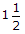times their heightsD.
 extending about one metre beyond a line drawn at an inclination of 2 : 1 to the axis of main canalE.
 all the above.18.

If the height of the hydraulic gradient line above the floor of thickness t is h and the specific gravity of the material of the floor is G, the minimum thickness t of the floor down stream of the crestwall, is given by the equation

A.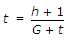B.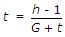C.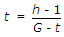D.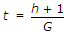19.

The ratio of the rate of change of discharge of an outlet to the rate of change in level of water surface in a distributary at its normal depth, is known as

A.
 efficiencyB.
 sensitivityC.
 flexibilityD.
 modular limit.20.

Lacey's equation for calculating flood discharge in rivers, is

A.
 V = 10.8 R1/2 S1/2B.
 V = 10.8 R2/3 S1/2C.
 V = 10.8 R2/3 S1/3D.
 V = 10.8 R1/3 S2/3